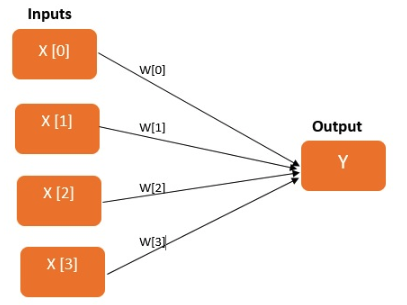# Bayesian Neural Network

Back to glossary

## What Are Bayesian Neural Networks?

Bayesian Neural Networks (BNNs) refers to extending standard networks with posterior inference in order to control over-fitting. From a broader perspective, the Bayesian approach uses the statistical methodology so that everything has a probability distribution attached to it, including model parameters (weights and biases in neural networks). In programming languages, variables that can take a specific value will turn the same result every-time you access that specific variable. Let’s begin with the revision of a simple linear model, which will predict the output by the weighted sum of a series of input features.In comparison, in the Bayesian world, you can have similar entities also known as random variables that will give you a different value every time you access it.  In Bayesian terms, the historical data represents our prior knowledge of the overall behavior with each variable having its own statistical properties which vary with time. Let’s assume that X is a random variable which represents the normal distribution, every time X gets accessed, the returned result will have different values This process of getting a new value from a random variable is called sampling. What value comes out depends on the random variable’s associated probability distribution. That means, in the parameter space, one can deduce the nature and shape of the neural network's learned parameters. Recently there has been a lot of activity in this area, with the advent of numerous probabilistic programming libraries such as PyMC3, Edward, Stan etc. Bayesian methods are used in lots of fields: from game development to drug discovery.

## What Are Some of the Main Advantages of BNNs?

• Bayesian neural nets are useful for solving problems in domains where data is scarce, as a way to prevent overfitting. Example applications are molecular biology and medical diagnosis (areas where data often come from costly and difficult experimental work).
• Bayesian nets are universally useful
• They can obtain better results for a vast number of tasks however they are extremely difficult to scale to large problems.
• BNNs allow you to automatically calculate an error associated with your predictions when dealing with data of unknown targets.
• allow you to estimate uncertainty in predictions, which is a great feature for fields like medicine

## Why should you use Bayesian Neural Networks?

Instead of taking into account a single answer to one question, Bayesian methods allow you to consider an entire distribution of answers. With this approach, you can naturally address issues such as:
• regularization (overfitting or not),
• model selection/comparison,without the need for a separate cross-validation data set